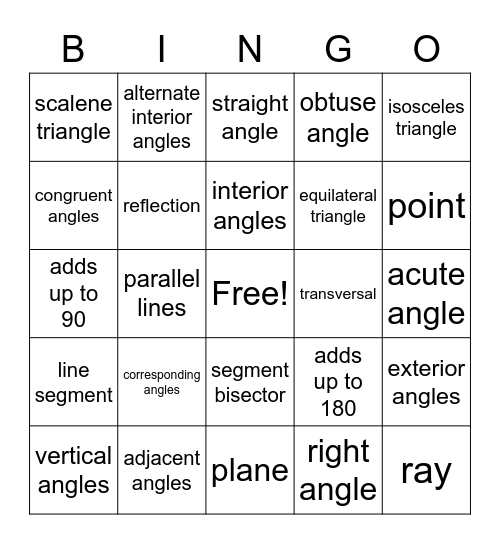# Geometry reviewThis bingo card has a free space and 24 words: corresponding angles, adjacent angles, interior angles, vertical angles, isosceles triangle, scalene triangle, congruent angles, alternate interior angles, transversal, segment bisector, equilateral triangle, adds up to 90, adds up to 180, exterior angles, reflection, right angle, acute angle, straight angle, point, parallel lines, ray, line segment, obtuse angle and plane.

## Play Online